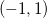# Bug in MATLAB – atanh gives incorrect result

by on September 21, 2011

Inverse hyperbolic tangent function `atanh()` in MATLAB gives incorrect results for arguments outside:

```>> atanh(-2)   ans =   -0.549306144334055 - 1.5707963267949i```

Imaginary part of the result has incorrect sign. Function `acoth()` is also affected by this bug (since it is calculated through `atanh()`). Tested up to MATLAB 2011a.

Multiprecision Computing Toolbox

```>> mp('atanh(-2)',30) -0.549306144334054845697622618461 + 1.57079632679489661923132169164i   >> atanh(mp('-2',30)) -0.549306144334054845697622618461 + 1.57079632679489661923132169164i```

Maple

```> arctanh(-2.0);
-0.5493061443 + 1.570796327 I
```

```atanh(-2) =